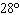Name:    Geometry Chapter 2 Practice MC Test

1.

Rewrite the statement in if-then form.
Every right triangle has an angle with a measure of 90 degrees.
 a. If a figure is a right triangle, then it has an angle with a measure of 90 degrees. b. A figure has an angle with a measure of 90 degrees if and only if it is a right triangle. c. If a figure has an angle with a measure of 90 degrees, then it is a right triangle. d. A figure is a right triangle if and only if it has an angle with a measure of 90 degrees.

2.

"If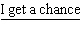, I will succeed." In this conditional statement, the underlined portion is ________.
 a. the conclusion b. the hypothesis c. the argument d. the converse

3.

The figure below represents which of the following statements?a. Two lines that are perpendicular b. AB = AC c. Two rays that are perpendicular d. A straight angle

4.

Given that:
i. Some students are intelligent..
ii. All intelligent people are snobs..
What conclusion can be logically deduced?
 a. All snobs are intelligent. c. Some students are snobs. b. All students are snobs. d. Some intelligent people are snobs.

Choose the phrase that completes the following statement as stated by the Point, Line, and Plane Postulates:

5.

One plane ___ passes through three noncollinear points.
 a. sometimes b. Point, Line, and Plane Postulates do not address this topic directly. c. never d. always

6.

Decide which one of the following statements is false.
 a. Any three points lie on a distinct line. b. A line contains at least two points. c. Three noncollinear points determine a plane. d. Through any two distinct points there exists exactly one line.

7.

Which of the following is an example of the Symmetric Property?
 a.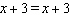b. If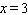, then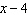=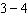. c. If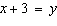and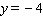, then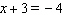. d. If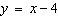, then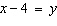.

8.

If PQ = 3 and PQ + RS = 5, then 3 + RS = 5 is an example of the __________.
 a. Transitive Property of Equality b. Substitution Property of Equality c. Reflexive Property of Equality d. Multiplication Property of Equality

9.

Identify the property of congruence.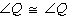.
 a. Reflexive Property of Congruence b. Transitive Property of Congruence c. Symmetric Property of Congruence d. Substitution Property of Equality

10.

In the figure shown,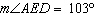. Which of the following statements is false?a.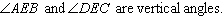b.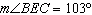c.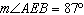d.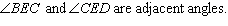11.

Solve for x.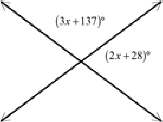a. 1 c. 6 b. 3 d. 2

12.

Give the reason for the last statement in the proof.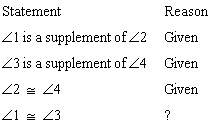a. Congruent Supplements Theorem b. Linear Pair Postulate c. Congruent Complements Theorem d. Vertical Angles Congruence Theorem

13.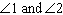form a linear pair. If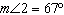, what is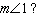a.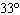c.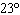b.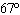d.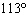14.are supplementary angles.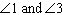are vertical angles. If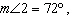what is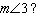a.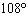c.b.d.